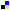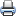# WDM Network DesignSaveDiggDel.icio.usPrint

### Chapter Description

This sample chapter explores some of the design constraints involved in the WDM network design.

## Calculation of Q-Factor from OSNR

The OSNR is the most important parameter that is associated with a given optical signal. It is a measurable (practical) quantity for a given network, and it can be calculated from the given system parameters. The following sections show you how to calculate OSNR. This section discusses the relationship of OSNR to the Q-factor.

The logarithmic value of Q (in dB) is related to the OSNR by Equation 4-11.

#### Equation 4-11In the equation, B0 is the optical bandwidth of the end device (photodetector) and Bc is the electrical bandwidth of the receiver filter.

Therefore, Q(dB) is shown in Equation 4-12.

#### Equation 4-12In other words, Q is somewhat proportional to the OSNR. Generally, noise calculations are performed by optical spectrum analyzers (OSAs) or sampling oscilloscopes, and these measurements are carried over a particular measuring range of Bm. Typically, Bm is approximately 0.1 nm or 12.5 GHz for a given OSA. From Equation 4-12, showing Q in dB in terms of OSNR, it can be understood that if B0 < Bc, then OSNR (dB )> Q (dB). For practical designs OSNR(dB) > Q(dB), by at least 1–2 dB. Typically, while designing a high-bit rate system, the margin at the receiver is approximately 2 dB, such that Q is about 2 dB smaller than OSNR (dB).

### Calculation of OSNR for a Point-to-Point Link

Consider a physical link AB, as shown in Figure 4-5. Assume this to be a long-haul fiber WDM link (a link that is several hundred kilometers). Amplifiers are placed periodically at repeated intervals to boost signal power. Therefore, a signal can reach much farther than the maximum allowable accumulated loss due to the fiber (υL). However, in doing so, each amplifier stage adds its own component of amplified spontaneous emission (ASE) noise and degrades the OSNR further. Moreover, every amplifier amplifies the already present noise. Note that noise is omnipresent throughout the spectra and almost impossible to be removed. Therefore, it is imperative to devise a method to calculate the OSNR (output) at the end of an N stage-amplified system and see if the value N is still valid.Figure 4-5 A Multiple Stage Amplified DWDM System Deployed in a Point-to-Point Topology

In an OSNR-based design, we must ensure that OSNR of the final stage is in compliance with system OSNR requirements and hence the BER requirements. To make the system support a particular BER, it is necessary to make the OSNR system design compliant.

The OSNR of each stage is shown in Equation 4-13.

#### Equation 4-13In Equation 4-13, NFstage is the noise figure of the stage, h is Plank's constant (6.6260 × 10-34), ν is the optical frequency 193 THz, and Δf is the bandwidth that measures the NF (it is usually 0.1 nm).

The total OSNR for the system can be considered by a reciprocal method and is shown in Equation 4-14.

#### Equation 4-14for the 'N' stage system. That summarizes to Equation 4-15.

#### Equation 4-15A slight detailed analysis provides a more appropriate equation for OSNR. For a single amplifier of gain G, the OSNR is shown in Equation 4-16.

#### Equation 4-16In Equation 4-16, nsp is the population inversion parameter that is shown in Equation 4-17 and is the ratio of electrons in higher and lower states.

#### Equation 4-17In Equation 4-17, N2 is the number of electrons in a higher state and N1 is the number of electrons in the lower state. (Refer to Chapter 2 for more details.)

The population inversion parameter is also shown in Equation 4-18.

#### Equation 4-18For an N amplifier stage system, with each amplifier compensating for the loss of the previous span where the span loss in dB is, you have the relationship for final stage OSNR in Equation 4-19.

#### Equation 4-19Taking logarithm to the common base (10), we get Equation 4-20.

#### Equation 4-20From the previous section, we get Δf = 0.1 nm, or 12.5 GHz. Substituting this, we get Equation 4-21.

#### Equation 4-21The following is assumed:

• The NF of every amplifier is the same. (we assume uniformity of products; therefore, NFs are the same for all amplifiers.)

•is the span loss and is same. (This is a generic assumption and can be changed, as shown later in this section.)

• Noise is totaled over both states of polarization. In short, it is unpolarized noise.

Equation 4-21 provides the actual mathematical calculation of OSNR. This calculation method has quite a few approximations in which we can still find the system OSNR to a great degree of accuracy. In a multichannel WDM system, the design should consider OSNR for the worst channel (the one that has the worst impairment). The worst channel is generally the first or last channel in the spectrum.

### OSNR Improvements by Raman Amplification

If we look at Equation 4-21, we can see that the EDFA gain factor G is not considered. That is because OSNR is a ratio, and the gain acts equally on signal and noise, canceling the gain factor in the numerator and denominator. In other words, although EDFAs alleviate the upper bound on transmission length due to attenuation, by cascading EDFAs in a series, the OSNR is continuously degraded with transmission length and ASE (from EDFAs). This degradation can be lessened somewhat by distributed Raman amplifiers (DRAs). As can be seen from Chapter 3, "Networking with DWDM -2," Raman amplification is inherently a result of stimulated Raman scattering of a high intensity pump signal at a different frequency (compared to the signal frequency). This produces a gain because of creation of a Stokes wave, which in turn produces a gain feeding wave of a wide bandwidth.

Consider a hybrid system as shown in Figure 4-6.Figure 4-6 Multistage Raman Amplifier-Based System

From the preceding system, the OSNR of the final stage is shown as in Equation 4-22.

#### Equation 4-22Equation 4-23 gives the OSNR value of each stage.

#### Equation 4-23As we can see from Equation 4-23, the factor GRA in the numerator actually enhances the OSNR of the system. Figure 4-7 shows the variation of Raman gain with pump power.Figure 4-7 Variation of Raman Gain with Pump Power

6. Margin Requirements | Next Section Previous Section